# Methods and formulas for the goodness-of-fit statistics in Simple Binary Logistic Regression

Select the method or formula of your choice.

## Deviance

Deviance measures the discrepancy between the current model and the full model. The full model is the model that has n parameters, one parameter per observation. The full model maximizes the log-likelihood function. The full model provides a point of comparison for models with fewer than n parameters. Comparisons to the full model use the scaled deviance.

The contribution to the scaled deviance from each individual data point depends on the model.

Model Deviance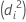Binomial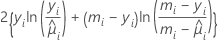Poisson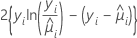The degrees of freedom for the test depend on the sample size and the number of terms in the model:

### Notation

TermDescription
Lf the log-likelihood for the full model
Lcthe log-likelihood of the model with a subset of terms from the full model
yi the number of events for the ith row in the datathe estimated mean response for the ith row in the data
mithe number of trials for the ith row in the data
nthe number of rows in the data
pthe regression degrees of freedom

## Pearson residuals

Elements of the Pearson chi-square that can be used to detect ill-fitted factor/covariate patterns. Minitab stores the Pearson residual for the ith factor/covariate pattern. The formula is:

### Notation

TermDescription
yithe response value for the ith factor/covariate patternthe fitted value for the ith factor/covariate pattern
Vthe variance function for the model atThe variance function depends on the model:

 Model Variance function Binomial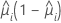Poisson## Hosmer-Lemeshow

A goodness-of-fit test for models with binary responses based on grouping data based on the estimated probabilities. It is the chi-square statistic from a 2 × g table of observed and estimated expected frequencies, where g is the number of groups. The degrees of freedom for the test is g − 2.

The formula is:

To form the groups, Minitab orders the estimated probabilities and then attempts to create 10 groups of equal size.

The expected number of events in a group is:

expected events =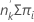The expected value for the number of nonevents is:

expected nonevents =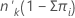### Notation

TermDescription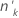The number of trials in the kth group
ok The number of events among thefactor/covariate patterns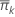The average estimated probability for each group
πi The fitted probabilities for the factor/covariate patterns in a group
By using this site you agree to the use of cookies for analytics and personalized content.  Read our policy